Instant Message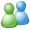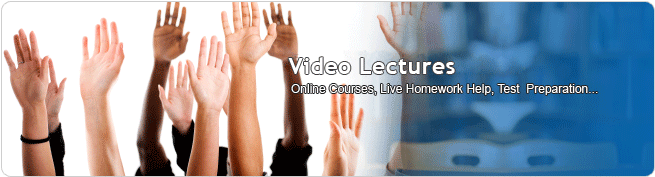Solution Library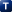: Sub Topics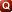: Questions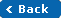## 20 Solution(s) found.

 Set theoryProbability Theory1 2
Question:  A uniform die is thrown at random. Find the probability that the number on it is (a) 4 (b) Greater than 4 (c) Even
Question:  The letters in the word article are arranged at random. Find the probability that the vowels may occupy odd places
Question:  If 6 dice are thrown then the what is the probability of all showing different faces
Question:  If 10 men among whom are A and B, stand in a row what is the probability that there will be exactly 3 men between them.
Question:  In a random arrangement of the letters of the word MATHEMATICS find the probability that all the vowels come together.
Question:  The letters in the word failure are arranged at random. Find the probability that the vowels may occupy even places
Question:  In a single throw of two uniform die. Find the probability of throwing (i) 4 (ii) 8
Question:  What is the probability that a non leap year should have fifty three Sundays?
Question:  Find the probability that in 5 tossings, a perfect coin turns up head atleast 3 times in succession
Question:  A bag contains 20 coupons marked with numbers 1-20. One coupon is drawn at random. Find the probability that it will be a multiple of (i) 2 or 5 (ii) 3 or 5
Question:  There are 4 clubs on a street. If 3 men walk into the clubs what is the probability they party in different clubs.
Question:  In a village of 21 inhabitants a person tells a rumour to a second person, who in turn repeats it to a third person, etc. at each step the recipient of the rumor is chosen at random from 20 available people. Find the probability that the rumour will be told 10 times without (i) returning to the originator (ii) being repeated to any person.
Question:  P(A bar)= 1-P(A)
Question:  Theorem: the probability of simultaneous happening of two events A and B is given by P(AnB)= P(A)P(B|A); P(A)=/=0 and P(BnA)= P(B)P(A|B); P(A)=/=0
Question:  Multiplication theorem for independent events: two events A and B are independent iff P(AnB)= P(A).P(B)
Question:  Introdction to sets
Question:  Some results on Sets
Question:  What are Disjoint sets?
Question:  Operations on Sets
Question:  n(A)=17 n(B)= 23, find n(Aintersection B)
 1 2